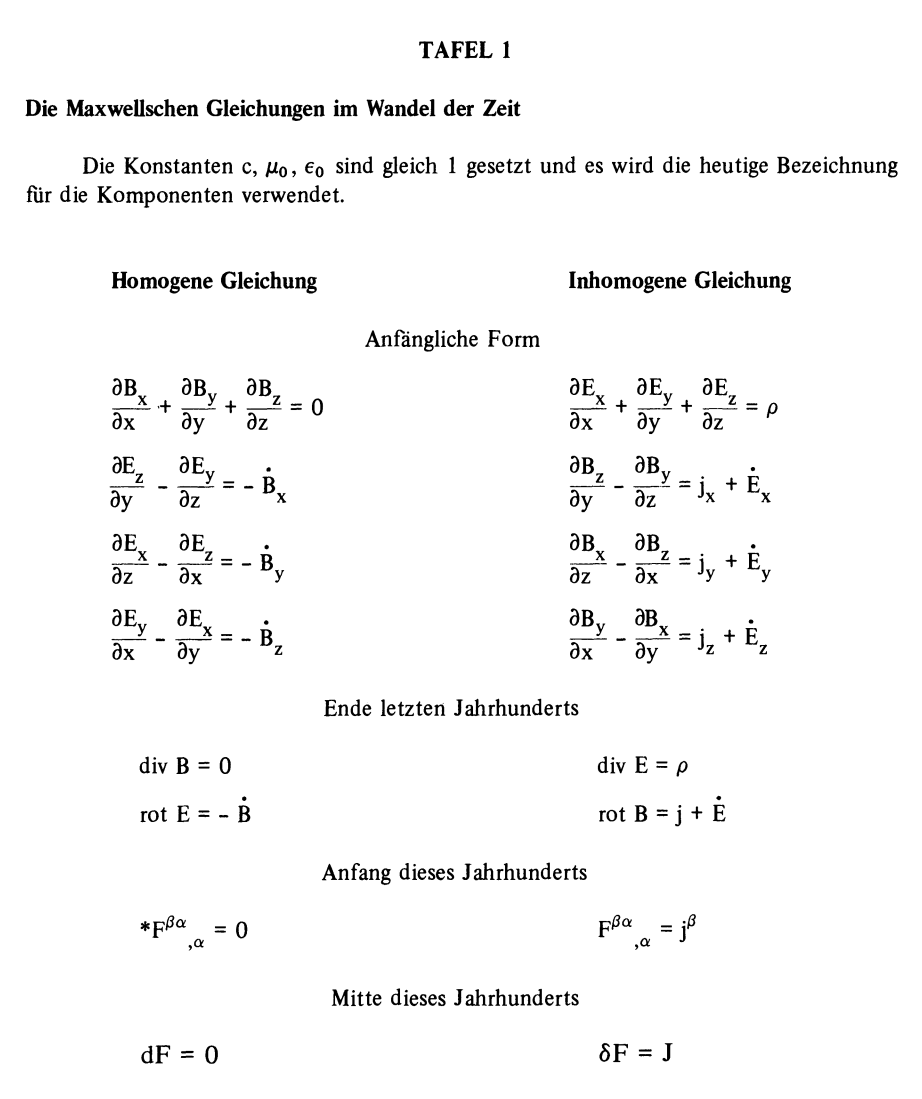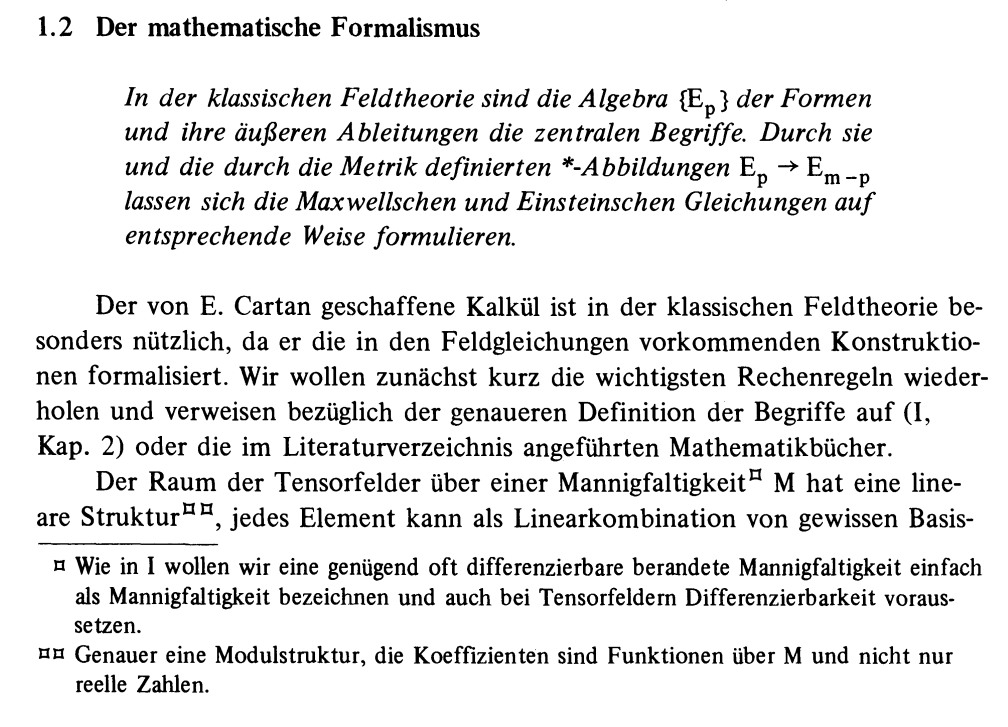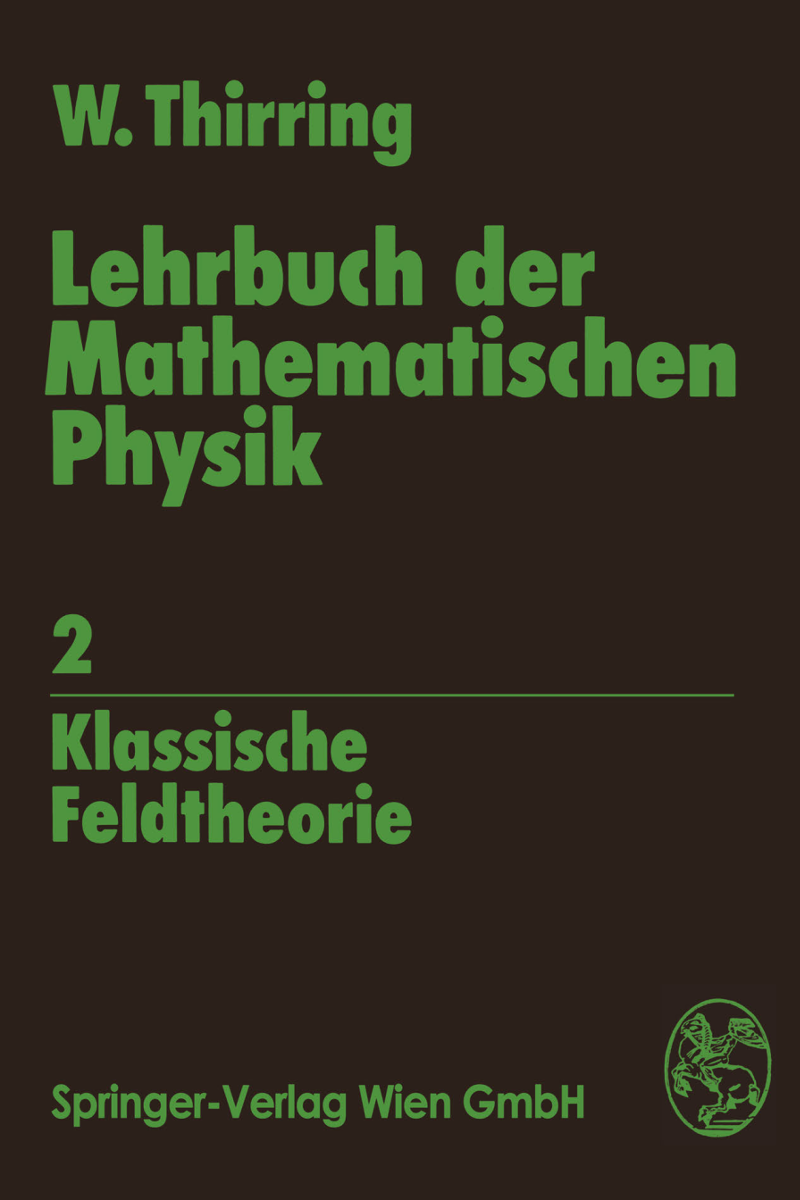# Evolution of Maxwell's equations

A great textbook in mathematical physics is the series of Thirring. I liked as a student especially the great summaries for each chapter (but I found the book very hard to digest). In any way, there is one great page in the second volume, which shows the evolution of the Maxwell equations. Obviously, we have learned to make things more elegant since Maxwell discovered the equations. I have only the German edition, but there is an English translation. Here is an example of such a section summary. We translate that summary:
 In classical field theories, the algebra Ep of forms and their exterior derivatives play a central role. Through them as well as through the *-map Ep -> Em-p one can write down the Maxwell and Einstein equations.